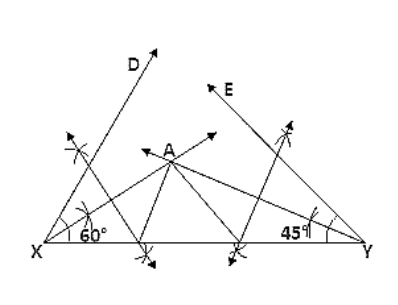# Construct a triangle whose perimeter is 6.4 cm,Question:

Construct a triangle whose perimeter is 6.4 cm, and angles at the base are 60° & 45°.

Solution:

Steps of Construction:

1. Draw a line segment XY of 6.4 cm.

2. Draw ∠DXY = 60° and ∠EYX = 45°.

3. Draw the angle bisectors of ∠DXY and ∠EYX which intersect each other at A.

4. Draw the perpendicular bisector of AX and AY which intersect XY at B and C respectively.

5. Join AB and AC.

Hence △ABC is the required triangle.